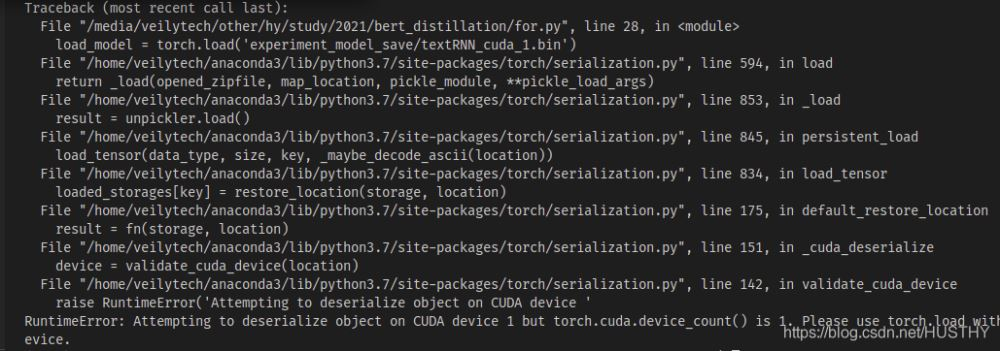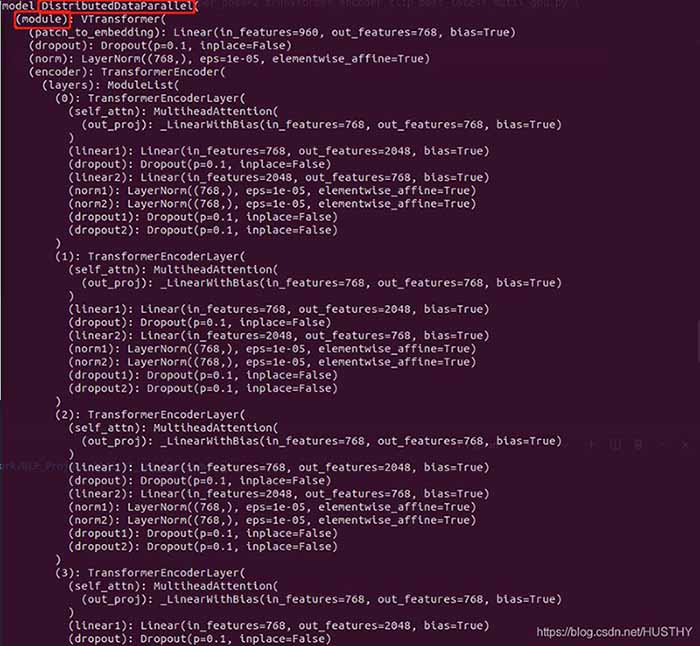﻿ pytorch模型保存与加载中的一些问题实战记录_python_脚本之家
python# pytorch模型保存与加载中的一些问题实战记录

## 一、torch中模型保存和加载的方式

### 1、模型参数和模型结构保存和加载

```torch.save(model,path)

### 2、只保存模型的参数和加载——这种方式比较安全，但是比较稍微麻烦一点点

```torch.save(model.state_dict(),path)

## 二、torch中模型保存和加载出现的问题

### 1、单卡模型下保存模型结构和参数后加载出现的问题```import torch
from model.TextRNN import TextRNN

### 2、多卡机器单卡训练模型保存后在单卡机器上加载会报错

```import torch
from model.TextRNN import TextRNN`load_model = torch.load('experiment_model_save/textRNN_cuda_1.bin',map_location=torch.device('cpu'))`

### 3、多卡训练模型保存模型结构和参数后加载出现的问题

a、模型结构和参数一起保然后在加载`torch.distributed.init_process_group(backend='nccl')`

b、单独保存模型参数

```model = Transformer(num_encoder_layers=6,num_decoder_layers=6)```model = torch.load('train_model/clip/Vtransformers_bert_6_layers_encoder_clip.bin')
print(model)
model.cuda(args.local_rank)
。。。。。。
model = nn.parallel.DistributedDataParallel(model,device_ids=[args.local_rank],find_unused_parameters=True)
print('model',model)```## 三、正确的保存模型和加载的方法

```    if gpu_count > 1:
torch.save(model.module.state_dict(),save_path)
else:
torch.save(model.state_dict(),save_path)
model = Transformer(num_encoder_layers=6,num_decoder_layers=6)